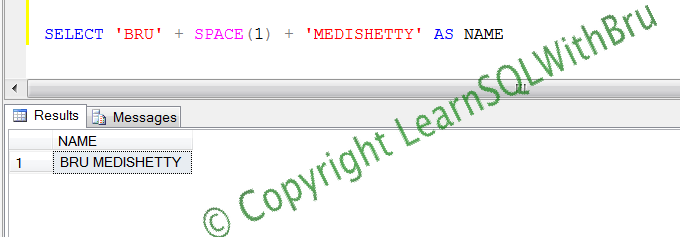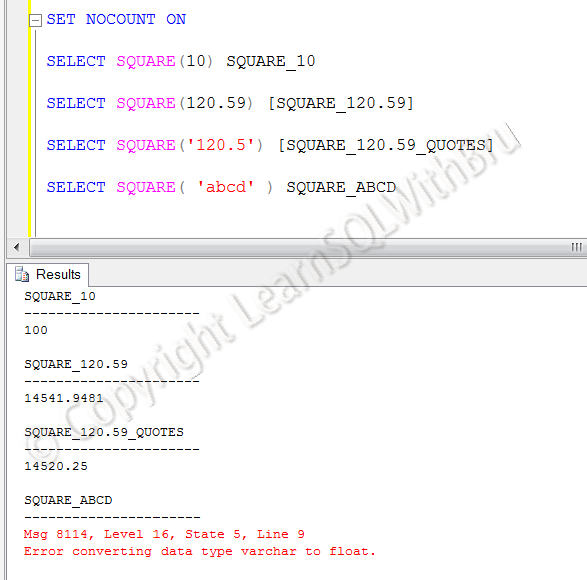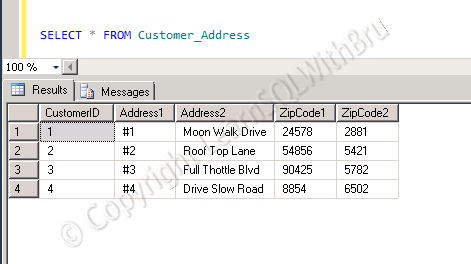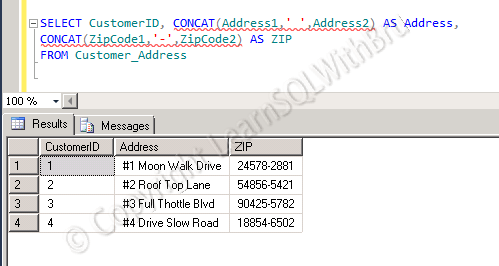# Space function in SQL Server

Few weeks back, I wrote a blog on CONCAT string function in SQL Server 2012. A user asked if the CONCAT function is similar to Space function in SQL Server and what is the difference between the two functions. I replied to him and clarified the difference of the 2 functions. After that I thought, it would be good to write a blog post on Space function too.

Space function

Space function is useful to return ‘x’ number of spaces, where ‘x’ is an integer.  The syntax for the space function is space(x) where x is an integer. To understand the function lets us look a simple script with and without space function.

Without Space function

The below screen shot displays an output where 2 string values are concatenated without using space function and the resulting string is an output that may not be acceptable. See that the resulting string is a single string without any break between first and last name.Using Space function

The below screen shot displays an output where 2 string values are concatenated and separated by space function. Using space(1) generated a single space (1 space) and when that is used in the string concatenation, output string is now better and acceptable.Do you like this site? Like our FB page @ Facebook.com\LearnSQLWithBru to know when there is new content.

— Bru Medishetty

# Square – Mathematical function in SQL Server

In this blog post we shall learn a mathematical function in SQL Server called Square. This function expects 1 value to be passed and returns the multiplied value itself nothing but the square of the passed value.

The function expects a value of float data type to be passed. Any other data types, such as an integer will be implicitly converted into a float value. See that the function treats 10 (integer) and 120.59 (float) as float and returns the expected square values..

If the passed value is a integer or a float value with quotes, then that is also converted into a float. See that the function converts ‘120.5’ to float 120.5 without issues.

When the value passed is a datetime or string characters (a – z) or special characters, the could not be converted to float, the function returns an error. See the error below, where ‘abcd’ is passed to the function.Do you like this site? Like our FB page @ Facebook.com\LearnSQLWithBru so that, you know when there is a new blog post.

— Bru Medishetty

# CONCAT – New String Function in SQL Server 2012

CONCAT is a new String function introduced in SQL Server 2012. This function returns an output which is a concatenated string value of the argument values passed in the function. The function would need a minimum of 2 values to be passed.

Let’s take a look at the function. In order to use this function, we shall first create a table and insert few records. The following script is used to the task of creating a table and inserting the data into that table.

```CREATE TABLE [dbo].[Customer_Address](
[CustomerID] [bigint] NULL,
[ZipCode1] [char](5) NULL,
[ZipCode2] [char](4) NULL
)

VALUES
(1,'#1','Moon Walk Drive', 24578,2881 ),
(2,'#2','Roof Top Lane', 54856,5421 ),
(3,'#3','Full Thottle Blvd', 90425,5782 ),

First let us look at the data in the table by doing a Select * on the table. The pic below displays the result set.Now let us run the following query which uses the CONCAT function to concatenate the Address1 ad Address2 columns as a single column and also concatenate the 5 character length ZipCode1 and 4 character length Zipcode2 and display a single column output. Note that I am going to use a space ‘ ‘, and a hyphen ‘-‘ in order to display the concatenated column in a meaningful way.

```SELECT CustomerID, CONCAT(Address1,' ',Address2) AS Address,
CONCAT(ZipCode1,'-',ZipCode2) AS ZIPIn previous Versions of SQL Server, you could concatenate string values using a +. If order to achieve the same result you can write the following code.
```SELECT CustomerID, Address1 + ' ' + Address2 AS Address,